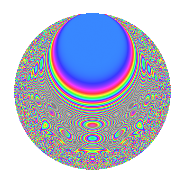# Properties

 Label 8027.2.a.dLevel 8027 Weight 2 Character orbit 8027.a Self dual Yes Analytic conductor 64.096 Analytic rank 1 Dimension 149 CM No

# Related objects

## Newspace parameters

 Level: $$N$$ = $$8027 = 23 \cdot 349$$ Weight: $$k$$ = $$2$$ Character orbit: $$[\chi]$$ = 8027.a (trivial)

## Newform invariants

 Self dual: Yes Analytic conductor: $$64.0959177025$$ Analytic rank: $$1$$ Dimension: $$149$$ Fricke sign: $$1$$ Sato-Tate group: $\mathrm{SU}(2)$

## $q$-expansion

The dimension is sufficiently large that we do not compute an algebraic $$q$$-expansion, but we have computed the trace expansion.

 $$\operatorname{Tr}(f)(q) =$$ $$149q$$ $$\mathstrut -\mathstrut 5q^{2}$$ $$\mathstrut -\mathstrut 5q^{3}$$ $$\mathstrut +\mathstrut 135q^{4}$$ $$\mathstrut -\mathstrut 28q^{5}$$ $$\mathstrut -\mathstrut 5q^{6}$$ $$\mathstrut -\mathstrut 33q^{7}$$ $$\mathstrut -\mathstrut 15q^{8}$$ $$\mathstrut +\mathstrut 134q^{9}$$ $$\mathstrut +\mathstrut O(q^{10})$$ $$\operatorname{Tr}(f)(q) =$$ $$149q$$ $$\mathstrut -\mathstrut 5q^{2}$$ $$\mathstrut -\mathstrut 5q^{3}$$ $$\mathstrut +\mathstrut 135q^{4}$$ $$\mathstrut -\mathstrut 28q^{5}$$ $$\mathstrut -\mathstrut 5q^{6}$$ $$\mathstrut -\mathstrut 33q^{7}$$ $$\mathstrut -\mathstrut 15q^{8}$$ $$\mathstrut +\mathstrut 134q^{9}$$ $$\mathstrut -\mathstrut 30q^{10}$$ $$\mathstrut -\mathstrut 20q^{11}$$ $$\mathstrut -\mathstrut 32q^{12}$$ $$\mathstrut -\mathstrut 73q^{13}$$ $$\mathstrut -\mathstrut 18q^{14}$$ $$\mathstrut -\mathstrut 43q^{15}$$ $$\mathstrut +\mathstrut 99q^{16}$$ $$\mathstrut -\mathstrut 32q^{17}$$ $$\mathstrut -\mathstrut 50q^{18}$$ $$\mathstrut -\mathstrut 32q^{19}$$ $$\mathstrut -\mathstrut 67q^{20}$$ $$\mathstrut -\mathstrut 36q^{21}$$ $$\mathstrut -\mathstrut 78q^{22}$$ $$\mathstrut -\mathstrut 149q^{23}$$ $$\mathstrut -\mathstrut 27q^{24}$$ $$\mathstrut +\mathstrut 75q^{25}$$ $$\mathstrut +\mathstrut 7q^{26}$$ $$\mathstrut -\mathstrut 23q^{27}$$ $$\mathstrut -\mathstrut 90q^{28}$$ $$\mathstrut -\mathstrut 20q^{29}$$ $$\mathstrut -\mathstrut 12q^{30}$$ $$\mathstrut -\mathstrut 34q^{31}$$ $$\mathstrut -\mathstrut 35q^{32}$$ $$\mathstrut -\mathstrut 63q^{33}$$ $$\mathstrut -\mathstrut 43q^{34}$$ $$\mathstrut +\mathstrut 24q^{35}$$ $$\mathstrut +\mathstrut 98q^{36}$$ $$\mathstrut -\mathstrut 228q^{37}$$ $$\mathstrut -\mathstrut 25q^{38}$$ $$\mathstrut -\mathstrut 19q^{39}$$ $$\mathstrut -\mathstrut 79q^{40}$$ $$\mathstrut -\mathstrut 4q^{41}$$ $$\mathstrut -\mathstrut 88q^{42}$$ $$\mathstrut -\mathstrut 70q^{43}$$ $$\mathstrut -\mathstrut 80q^{44}$$ $$\mathstrut -\mathstrut 153q^{45}$$ $$\mathstrut +\mathstrut 5q^{46}$$ $$\mathstrut -\mathstrut 3q^{47}$$ $$\mathstrut -\mathstrut 95q^{48}$$ $$\mathstrut +\mathstrut 86q^{49}$$ $$\mathstrut -\mathstrut 5q^{50}$$ $$\mathstrut -\mathstrut 57q^{51}$$ $$\mathstrut -\mathstrut 146q^{52}$$ $$\mathstrut -\mathstrut 110q^{53}$$ $$\mathstrut -\mathstrut 18q^{54}$$ $$\mathstrut -\mathstrut 33q^{55}$$ $$\mathstrut -\mathstrut 75q^{56}$$ $$\mathstrut -\mathstrut 132q^{57}$$ $$\mathstrut -\mathstrut 92q^{58}$$ $$\mathstrut +\mathstrut 41q^{59}$$ $$\mathstrut -\mathstrut 107q^{60}$$ $$\mathstrut -\mathstrut 82q^{61}$$ $$\mathstrut -\mathstrut 34q^{62}$$ $$\mathstrut -\mathstrut 99q^{63}$$ $$\mathstrut +\mathstrut 35q^{64}$$ $$\mathstrut -\mathstrut 47q^{65}$$ $$\mathstrut -\mathstrut 58q^{66}$$ $$\mathstrut -\mathstrut 162q^{67}$$ $$\mathstrut -\mathstrut 80q^{68}$$ $$\mathstrut +\mathstrut 5q^{69}$$ $$\mathstrut -\mathstrut 88q^{70}$$ $$\mathstrut -\mathstrut q^{71}$$ $$\mathstrut -\mathstrut 117q^{72}$$ $$\mathstrut -\mathstrut 124q^{73}$$ $$\mathstrut -\mathstrut 51q^{74}$$ $$\mathstrut -\mathstrut q^{75}$$ $$\mathstrut -\mathstrut 74q^{76}$$ $$\mathstrut -\mathstrut 56q^{77}$$ $$\mathstrut -\mathstrut 95q^{78}$$ $$\mathstrut -\mathstrut 89q^{79}$$ $$\mathstrut -\mathstrut 90q^{80}$$ $$\mathstrut +\mathstrut 93q^{81}$$ $$\mathstrut -\mathstrut 91q^{82}$$ $$\mathstrut -\mathstrut 64q^{83}$$ $$\mathstrut -\mathstrut 93q^{84}$$ $$\mathstrut -\mathstrut 155q^{85}$$ $$\mathstrut -\mathstrut 21q^{86}$$ $$\mathstrut -\mathstrut 49q^{87}$$ $$\mathstrut -\mathstrut 263q^{88}$$ $$\mathstrut -\mathstrut 60q^{89}$$ $$\mathstrut -\mathstrut 122q^{90}$$ $$\mathstrut -\mathstrut 130q^{91}$$ $$\mathstrut -\mathstrut 135q^{92}$$ $$\mathstrut -\mathstrut 179q^{93}$$ $$\mathstrut -\mathstrut 21q^{94}$$ $$\mathstrut +\mathstrut 30q^{95}$$ $$\mathstrut -\mathstrut 17q^{96}$$ $$\mathstrut -\mathstrut 199q^{97}$$ $$\mathstrut -\mathstrut 72q^{98}$$ $$\mathstrut -\mathstrut 91q^{99}$$ $$\mathstrut +\mathstrut O(q^{100})$$

## Embeddings

For each embedding $$\iota_m$$ of the coefficient field, the values $$\iota_m(a_n)$$ are shown below.

For more information on an embedded modular form you can click on its label.

Label $$a_{2}$$ $$a_{3}$$ $$a_{4}$$ $$a_{5}$$ $$a_{6}$$ $$a_{7}$$ $$a_{8}$$ $$a_{9}$$ $$a_{10}$$
1.1 −2.77862 1.96044 5.72072 −1.21161 −5.44733 1.22758 −10.3385 0.843341 3.36659
1.2 −2.75811 −1.16707 5.60719 −1.99614 3.21892 −2.42048 −9.94903 −1.63794 5.50558
1.3 −2.74408 1.08258 5.52999 4.29779 −2.97068 1.02479 −9.68658 −1.82803 −11.7935
1.4 −2.74361 −3.40606 5.52740 −0.590422 9.34489 −1.99716 −9.67782 8.60122 1.61989
1.5 −2.63043 0.0298964 4.91916 −2.77768 −0.0786403 −4.82248 −7.67864 −2.99911 7.30650
1.6 −2.60291 2.78039 4.77512 −1.36371 −7.23709 −4.03414 −7.22339 4.73056 3.54962
1.7 −2.59099 −0.493919 4.71322 1.04082 1.27974 −3.08433 −7.02991 −2.75604 −2.69675
1.8 −2.57108 2.45924 4.61046 0.658521 −6.32291 1.43884 −6.71170 3.04787 −1.69311
1.9 −2.55845 −2.16736 4.54566 2.09071 5.54507 −1.08828 −6.51294 1.69743 −5.34897
1.10 −2.55473 −3.25140 4.52663 2.74770 8.30644 1.12543 −6.45485 7.57160 −7.01962
1.11 −2.54629 −1.60651 4.48361 −2.62778 4.09064 1.52719 −6.32399 −0.419134 6.69110
1.12 −2.37150 0.0708005 3.62401 3.28290 −0.167903 −0.427363 −3.85135 −2.99499 −7.78539
1.13 −2.34386 0.672591 3.49366 0.169027 −1.57646 4.26981 −3.50093 −2.54762 −0.396175
1.14 −2.34025 −2.24282 3.47677 1.33607 5.24876 2.78387 −3.45602 2.03023 −3.12673
1.15 −2.33451 2.56074 3.44993 1.12501 −5.97807 −4.54116 −3.38489 3.55739 −2.62635
1.16 −2.31791 −1.39322 3.37270 −1.41282 3.22936 3.95674 −3.18178 −1.05893 3.27480
1.17 −2.27388 0.787573 3.17053 −4.28008 −1.79085 1.25028 −2.66164 −2.37973 9.73239
1.18 −2.26494 1.60784 3.12995 −1.10184 −3.64166 0.234772 −2.55928 −0.414855 2.49560
1.19 −2.21508 0.0631249 2.90657 −0.959004 −0.139827 −0.280080 −2.00812 −2.99602 2.12427
1.20 −2.20146 −2.87956 2.84641 0.0712627 6.33923 3.68192 −1.86333 5.29189 −0.156882
See next 80 embeddings (of 149 total)
 $$n$$: e.g. 2-40 or 990-1000 Embeddings: e.g. 1-3 or 1.149 Significant digits: Format: Complex embeddings Normalized embeddings Satake parameters Satake angles

## Inner twists

This newform does not have CM; other inner twists have not been computed.

## Atkin-Lehner signs

$$p$$ Sign
$$23$$ $$1$$
$$349$$ $$1$$

## Hecke kernels

This newform can be constructed as the kernel of the linear operator $$T_{2}^{149} + \cdots$$ acting on $$S_{2}^{\mathrm{new}}(\Gamma_0(8027))$$.